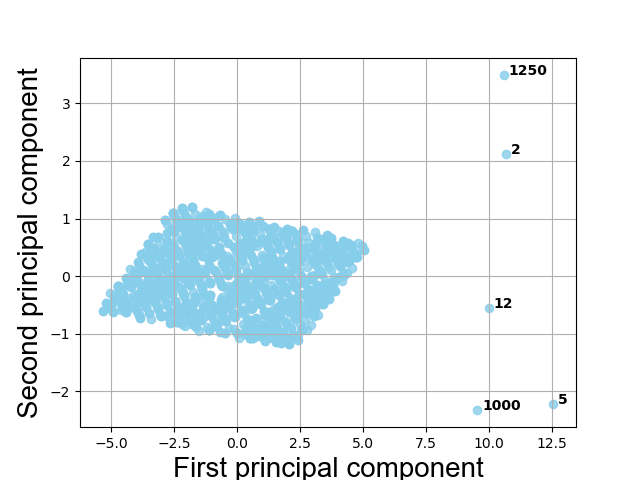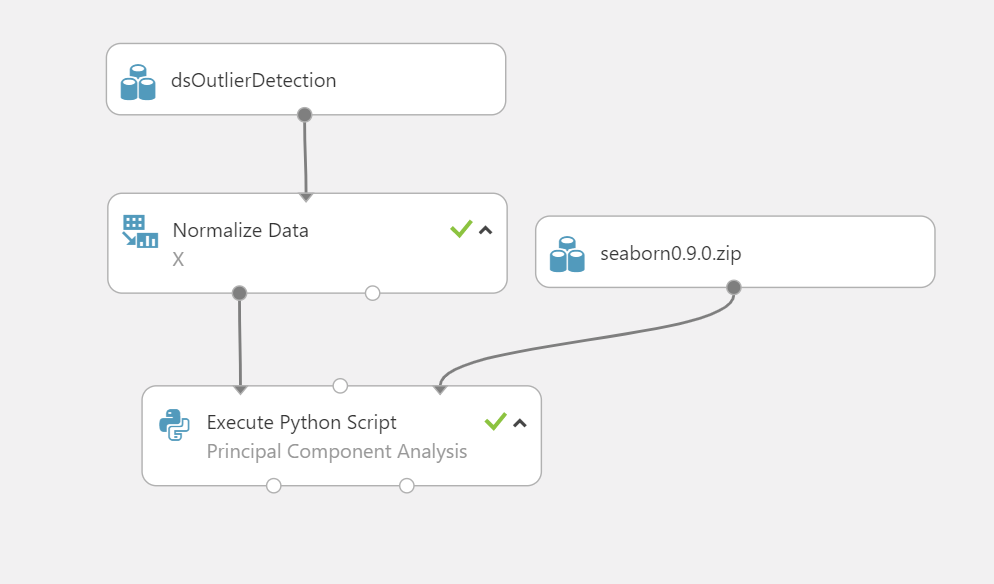# Outlier Detection using Principal Component Analysis

## Summary

My first article presents an analysis of an experiment on a structured dataset containing $$5$$ outliers. The goal of the experiment is to find the outliers, and the analysis is based on $$1,500$$ observations and $$7$$ features.

After standardization the data, a model of a set of linearly uncorrelated principal components was created.

After creating the linear model, a figure showing outliers in the dataset was created, and finally a table of statistics of the principal components was created.

## Nomenclature

\begin{array}{|l|l|}
\hline
\textbf{Symbol} & \textbf{Definition} \\
\hline
\mathbb{R} & \text{Set of real numbers}\\
\mu(\cdot) & \text{Mean}\\
||\cdot||_{2} & \text{Euclidean norm}\\
\sigma^{2}(\cdot) & \text{Variance}\\
m & \text{Number of observations in the experiment}\\
n & \text{Number of features in the experiment}\\
X: \mathbb{R}^{n} \rightarrow \mathbb{R}^{m} & m \times n \text{ design matrix}\\
\mathbf{x_{k}} \in \mathbb{R}^{m}& \text{The k-th feature vector in } X\\
L & \text{The number of principal components}\\
U: \mathbb{R}^{L} \rightarrow \mathbb{R}^{m} & m \times L \text{ matrix that contains the left singular }\\
&\text{orthogonal unit vectors of }X \text{ corresponding to}\\
& \text{the largest singular values}\\
\lambda_{k} & \text{The k-th eigenvalue of the covariance matrix } X^{T}X\\
\mathbf{u_{k}} \in \mathbb{R}^{m} & \text{The k-th left singular orthogonal unit vector of }X\\
\mathbf{c_{k}} \in \mathbb{R}^{m} & \text{The k-th principal component}\\
\mathcal{N}\left(\mu,\sigma^{2}\right) & \text{Normal distribution with mean } \mu \text{ and variance } \sigma^{2}\\
\hline
\end{array}

## Dataset

My dataset consists of $$n = 7$$ features and $$m = 1,500$$ observations. Each entry in the dataset contains random noise. The first $$5$$ observations are shown below:

\begin{array}{|r|r|r|r|r|r|r|}
\hline \mathbf{x_{1}}
& \mathbf{x_{2}}
& \mathbf{x_{3}}
& \mathbf{x_{4}}
& \mathbf{x_{5}}
& \mathbf{x_{6}}
& \mathbf{x_{7}}\\
\hline
63 & 55 & 59 & 57 & 67 & 52 & 70\\
64 & -2 & -3 & -4 & -5 & 51 & 74\\
65 & 55 & 60 & 57 & 71 & 51 & 76\\
69 & 59 & 64 & 61 & 75 & 55 & 79\\
-1 & 59 & -3 & -4 & -5 & 56 & 79\\
\hline
\end{array}

## Design Matrix

The design matrix $$X$$ is derived from the dataset by standardization. Because you do not want to compare apples and oranges, I decided to scale the dataset such that the features have the properties of a standard normal distibution ( $$\mu = 0$$ and $$\sigma^{2} = 1$$ ).  Thus for $$k \in \left\{1,2,3,4,5,6,7\right\}$$ holds $\mathbf{x_{k}}\sim \mathcal{N}\left(0,1\right)$

## Principal Component Analysis

The goal of the algorithm is to find uncorrelated principal components that retains most of the information. The algorithm uses the Singular Value Decomposition of $$X$$ to find the principal components :

The inputs of the algorithm are the components to keep $$L = 4$$ and the design matrix $$X$$.

The output is a linear transformation of $$X$$ in $$4$$ uncorrelated principal components.

## Model

The linear transformation of $$X$$ in $$4$$  uncorrelated principal components has the model below.$\begin{bmatrix}\mathbf{c_{1}}&\mathbf{c_{2}}&\mathbf{c_{3}}&\mathbf{c_{4}}\end{bmatrix} =\begin{bmatrix}97.14\mathbf{u_{1}}&22.55\mathbf{u_{2}}&17.62\mathbf{u_{3}}&9.30\mathbf{u_{4}}\end{bmatrix}=$
$U \begin{bmatrix} 97.14&0&0&0\\ 0&22.55&0&0\\ 0&0&17.62&0\\ 0&0&0&9.30\\ \end{bmatrix}$
where the matrix at the left-hand side contains the principal components, and at the right-hand side you see the diagonal matrix containing the singular values of $$X$$.

The figure below show you 5 numbered observations that can be considered as outliers.## Evaluation

Statistics are computed for each principal component. The results are shown in the table below.

\begin{array}{|c|r|r|r|}
\hline
k &\sigma^{2}\left(\mathbf{c_{k}}\right) &\sqrt{\lambda_{k}} &\mu\left(\mathbf{c_{k}}\right) \\
\hline
1 & 6.2907864 & 97.14 &0\\
2 & 0.3390017 & 22.55 &0\\
3 & 0.2067414 & 17.62 &0\\
4 & 0.0576600 & 09.30 &0\\\hline
\end{array}

Because of $$\mathbf{c_{1}}$$ has the highest variance, you may conclude that the first component retains most of the information.

Moreover, there holds that $$\lambda_{k} = 1,500\sigma^{2}\left(\mathbf{c_{k}}\right) \forall k \in \{1,2,3,4\}$$ due to the equation $n \sigma^{2}\left(\mathbf{c_{k}}\right) = ||\mathbf{c_{k}}||^{2}_{2} =\mathbf{c^{T}_{k}}\mathbf{c_{k}}=\sqrt{\lambda_{k}}\left(\mathbf{u^{T}_{k}}\mathbf{u_{k}}\right)\sqrt{\lambda_{k}} = \lambda_{k}.$

## Conclusion

The experiment was performed on a structured dataset and contains highly deviant observations in comparison with the other $$1,495$$ observations. This analysis has shown that the $$5$$ outliers can be confidently found using principal components.

## Machine Learning Experiment